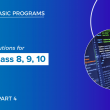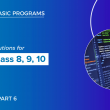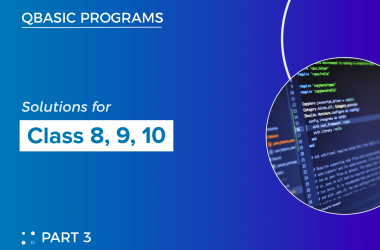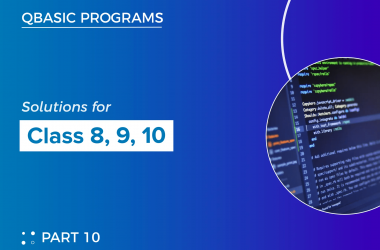# Qbasic programs solutions(part 5) for class 8, class 9 and class 10

Here, I have listed some of the qbasic programs. We also have mobile app where you can view all the qbasic programs. For better experience I highy recommend you to use our mobile app

81  Write a program to input any number and display whether it is odd or even.

``````    CLS
INPUT “ENTER ANY NUMBER”; N
IF N MOD 2 = 0 THEN
PRINT N; “IS EVEN NUMBER”
ELSE
PRINT N; “IS ODD NUMBER”
END IF
END ``````

82  Write a program to input any number and check whether the given no. is divisible by 5 or not.

``````CLS
INPUT “ENTER ANY NUMBER”; N
IF N MOD 5 = 0 THEN
PRINT N; IS DIVISIBLE BY 5”
ELSE
PRINT N; IS NOT DIVISIBLE BY 5”
END IF
END ``````

83  Write a program in qbasic to enter any 15 numbers and print only those numbers which are divisible by 5

``````    CLS
DIM N(15)
FOR I = 1 TO 15
INPUT "ENTER THE NUMBERS"; N(I)
NEXT I
PRINT "THE NUMBERS WHICH ARE DIVISIBLE BY 5 ARE"
FOR I = 1 TO 15
IF N(I) MOD 5 = 0 THEN PRINT N(I)
NEXT I
END ``````

84  Write a program in qbasic to generate the following series 9   28   14   7   22   11…………10th term

``````  CLS
A = 9
FOR I = 1 TO 10
PRINT A;
IF A MOD 2 = 0 THEN
A = A \ 2
ELSE
A = A * 3 + 1
END IF
NEXT I
END  ``````

85  Write a program in qbasic to ask number and count total number of odd digits

``````    REM
CLS
INPUT "ENTER ANY NUMBER"; N
C = 0
WHILE N <> 0
R = N MOD 10
IF R MOD 2 = 1 THEN C = C + 1
N = N \ 10
WEND
PRINT "TOTAL NUMBER OF ODD DIGITS"; C
END ``````

86  Write a program in qbasic to print square root of an ask number

``````REM PROGRAM TO DISPLAY SQUARE ROOT OF AN INPUT NUMBER
CLS
INPUT “ENTER ANY NUMBER”; N
S = N ^ (1 / 2)
PRINT “SQUARE ROOT OF NUMBER ”; S
END ``````

87  Write a program in qbasic to enter any ten strings and print the shortest string

``````    CLS
INPUT "ENTER FIRST STRING"; A\$
FOR I = 2 TO 10
INPUT "ENTER NEXT STRING"; B\$
IF LEN(B\$) < LEN(A\$) THEN A\$ = B\$
NEXT I
PRINT "SHORTEST STRING="; A\$
END ``````

88  Write a program to enter any two numbers and display the greater one.

``````   CLS
INPUT “ENTER ANY TWO NUMBERS”; A, B
IF A > B THEN
PRINT A; “IS GREATER”
ELSE
PRINT B; “IS GREATER”
END IF
END ``````

89   Write a program in qbasic to enter any 15 numbers and print only those numbers which are divisible by 5 and 7

``````    CLS
DIM N(15)
FOR I = 1 TO 15
INPUT "ENTER THE NUMBERS"; N(I)
NEXT I
PRINT "THE NUMBERS WHICH ARE DIVISIBLE BY 5 AND 7 ARE"
FOR I = 1 TO 15
IF N(I) MOD 5 = 0 AND N(I) MOD 7 = 0 THEN PRINT N(I)
NEXT I
END ``````

90  Write a program in qbasic to generate the following series 1   23   456   78910

``````    CLS
N = 1
FOR I = 1 TO 4
FOR J = 1 TO I
PRINT N;
N = N + 1
NEXT J
PRINT
NEXT I
END ``````

91  Write a program in qbasic to ask number and check whether the given no is prime or composite

``````    CLS
INPUT "ENTER ANY NUMBER"; N
C = 0
FOR I = 1 TO N
IF N MOD I = 0 THEN C = C + 1
NEXT I
IF C = 2 THEN
PRINT N; "IS PRIME NUMBER"
ELSE
PRINT N; "IS COMPOSITE NUMBER"
END IF
END ``````

92  Write a program in qbasic to convert binary number to decimal number

``````    CLS
INPUT "ENTER BINARY NUMBER"; N\$
FOR I = LEN(N\$) TO 1 STEP -1
B\$ = MID\$(N\$, I, 1)
S = S + VAL(B\$) * 2 ^ P
P = P + 1
NEXT I
PRINT "DECIMAL EQUIVALENT VALUE="; S
END ``````

93  Write a program in qbasic to ask any number and check whether the given number is Armstrong or not

``````    CLS
INPUT "ENTER ANY NUMBER"; N
A = N
S = 0
WHILE N <> 0
R = N MOD 10
S = S + R ^ 3
N = N \ 10
WEND
IF A = S THEN
PRINT A; "IS ARMSTRONG"
ELSE
PRINT A; "IS NOT ARMSTRONG"
END IF
END ``````

94  Write a program in qbasic to print first ten multiples of ask number

``````    CLS
INPUT “ENTER ANY NUMBER”; N
FOR I = 1 TO 10
PRINT N * I,
NEXT I
END
﻿``````

95  Write a program in qbasic to ask three sides of a triangle and determine whether a triangle is right angled triangle or not

``````    CLS
INPUT “ENTER HEIGHT, BASE AND PERPENDICULAR”; H, B, P
IF H ^ 2 = (B ^ 2 + P ^ 2) THEN
PRINT “IT IS A RIGHT ANGLED TRIANGLE”
ELSE
PRINT “IT IS NOT A RIGHT ANGLED TRIANGLE”
END IF
END ``````

96  Write a program in QBasic TO CHECK WHETHER THE ASK NUMBER IS PERFECT NUMBER OR NOT

``````    CLS
INPUT "ENTER ANY NUMBER"; N
S = 0
FOR I = 1 TO N - 1
IF N MOD I = 0 THEN S = S + I
NEXT I
IF S = N THEN
PRINT "PERFECT NUMBER"
ELSE
PRINT "NOT PERFECT NUMBER"
END IF
END ``````

97  Write a program in qbasic to print the sum of the numbers between 2 to 50

``````    CLS
FOR I = 2 TO 50
S = S + I
NEXT I
PRINT “SUM OF NUMBERS BETWEEN 2 TO 50” ; S
END  ``````

98  Write a program in qbasic to generate the following numeric pattern 1   12   123   1234   12345

``````    CLS
FOR I = 1 TO 5
FOR J = 1 TO I
PRINT J;
NEXT J
PRINT
NEXT I
END  ``````

99  Write a program in qbasic to check whether the supplied character is alphabet or not

``````    CLS
INPUT "ENTER ANY CHARACTER"; A\$
B = ASC(A\$)
IF B >= 65 AND B <= 90 OR B  >= 97 AND B <= 122 THEN
CK\$ = "SUPPLIED CHARACTER IS ALPHABET"
ELSE
CK\$ = "SUPPLIED CHARACTER IS NOT ALPHABET"
END IF
PRINT CK\$
END  ``````

##### Previous Post## Qbasic programs solutions(part 4) for class 8, class 9 and class 10

##### Next Post## Qbasic programs solutions(part 6) for class 8, class 9 and class 10

##### Related Posts## Qbasic Arrays with examples

QBasic is a programming language and integrated development environment (IDE) that was included with the Microsoft MS-DOS operating…## Qbasic programs solutions(part 3) for class 8, class 9 and class 10

Here, I have listed some of the qbasic programs. We also have mobile app where you can view…## Qbasic programs solutions(part 10) for class 8, class 9 and class 10

Here, I have listed some of the qbasic programs. We also have mobile app where you can view…